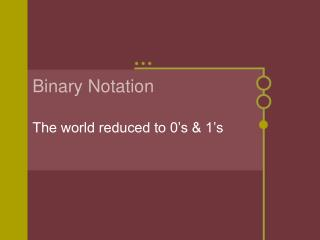DownloadDownload PresentationBinary Notation

# Binary Notation

Télécharger la présentation## Binary Notation

- - - - - - - - - - - - - - - - - - - - - - - - - - - E N D - - - - - - - - - - - - - - - - - - - - - - - - - - -
##### Presentation Transcript

1. Binary Notation The world reduced to 0’s & 1’s

2. What are binary numbers? • Decimal system uses ten symbols to represent numeric information • 0 1 2 3 4 5 6 7 8 9 • Each position within a number represents 10 raised to a power • Binary system uses two symbols to represent numeric information • 0 1 • Each position within a number represents 2 raised to a power • Binary numbers can be converted to decimal numbers and vice versa

3. Using binary numbers • Binary numbers can be used for mathematical operations • 101 + 110 = 1011 • Binary numbers can be used to represent characters • Morse Code - • S ... U ..- S ... A .- N -.

4. Binary symbol representation • Two numbers/symbols can be represented in many ways • 0 1 • On Off • True False • Yes No • Open Closed

5. Gottfried Wilhelm Liebniz (1646 - 1716) Logic from verbal state to absolute mathematical condition Influenced by ‘I Ching’ George Boole (1815 - 1864) Boolean Algebra Two Object On / Off, True / False Three Operations AND / OR / NOT Binary & Boolean Logic

6. How does Boolean Logic work?

7. Why do computers use binary? • Must have a way to represent data and instructions • Minimum representation uses two symbols • Binary is the smallest numbering system with only two symbols, 0 & 1 • Binary numbers combined with Boolean logic create circuits capable of mathematical and logic operations

8. Not every computer used binary The Eniac, Electronic Numerical Integrator and Computer, used a decimal system

9. Binary symbol representation There are five different ways to represent binary symbols within a computer Some volatile - some more permanent

10. Integrated Circuits • Electrical representation • Multiple transistors on silicon chip • Open - electricity can flow - 1 • Closed - no electrical flow - 0 • Volatile • CPU • Ram

11. Wire Connections • Electrical representation • 1.8 volts (or greater) - 1 • 0 volts - 0 • Volatile • System bus

12. Magnetic • Magnetic properties of iron oxide particles covering a surface • Particles in one direction - 1 • Particles in opposite direction - 0 • Non-volatile • Hard disk

13. Optical • Reflective surface with burned areas • Burned - Pit -1 • Not burned - Land - 0 • Non-volatile • CD • DVD

14. Solid State • Trapped electrons in a grid pattern with gates • Open - 1 • Closed - 0 • Non-volatile • Flash Drive

15. How binary notation represents data • Bit - binary digit • on / off, 1 / 0, true / false, open / closed • Byte - eight bits • Smallest unit for storing data

16. How binary notation represents data • Numeric data • Numbers in binary • Instructions • Coded patterns of 0’s & 1’s • Characters • Encoding systems

17. Encoding Systems

18. Who is behind encoding • Standards Organizations • The Unicode Consortium • American National Standards Institute - ANSI • Institute of Electrical and Electronics Engineers - IEEE • International Organization for Standardization - ISO

19. Using binary notation for other data • Analog signal • Continuous waves • Digital signal • Set of numbers • Can be used to describe a wave • Can also be used to recreate a wave